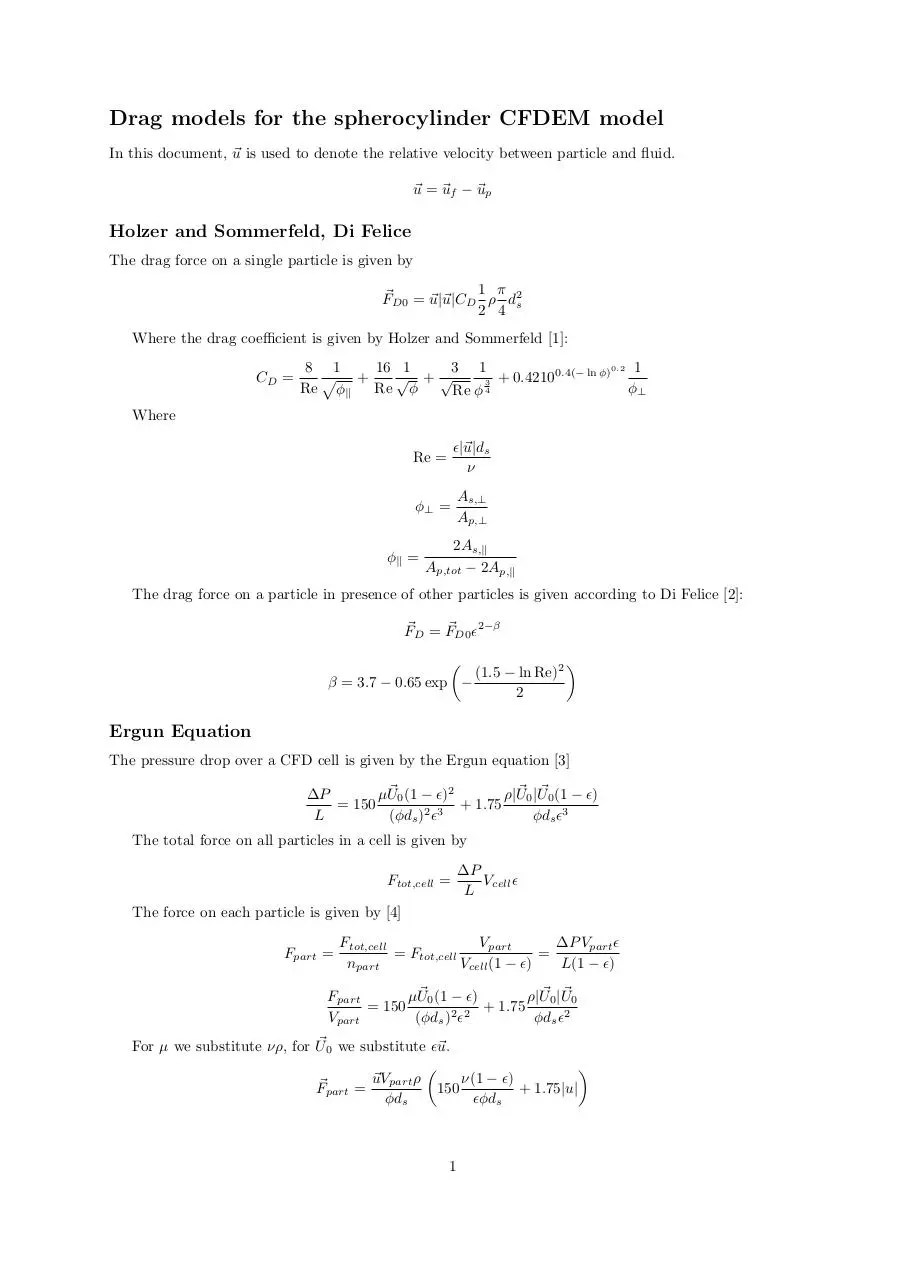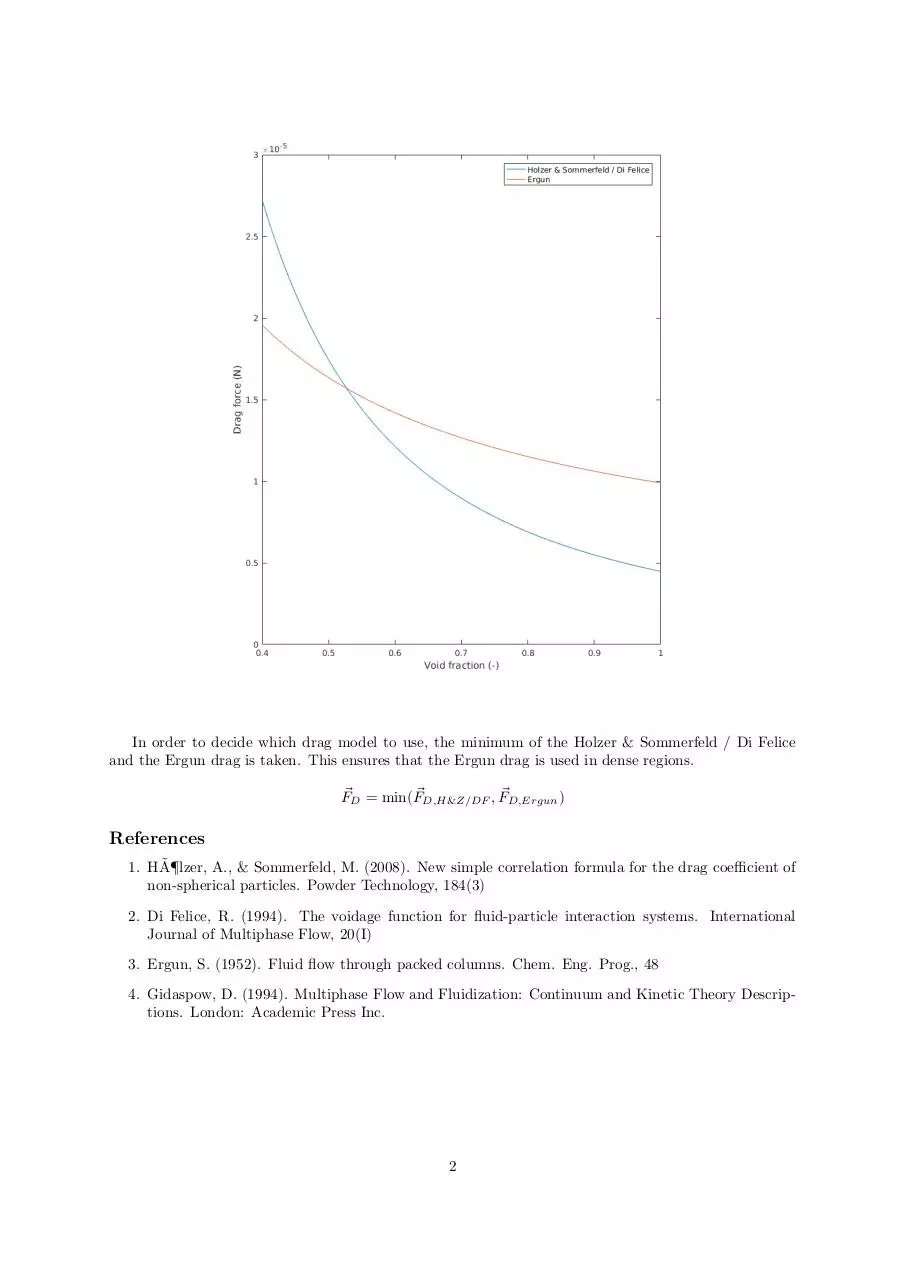# dragmodels .pdf

### File information

This PDF 1.5 document has been generated by TeX / MiKTeX pdfTeX-1.40.17, and has been sent on pdf-archive.com on 29/03/2017 at 14:54, from IP address 131.155.x.x. The current document download page has been viewed 282 times.
File size: 141.65 KB (2 pages).
Privacy: public file

### Document preview

Drag models for the spherocylinder CFDEM model
In this document, ~u is used to denote the relative velocity between particle and fluid.
~u = ~uf − ~up

Holzer and Sommerfeld, Di Felice
The drag force on a single particle is given by
1 π
F~D0 = ~u|~u|CD ρ d2s
2 4
Where the drag coefficient is given by Holzer and Sommerfeld :
CD =

16 1
3 1
8 1
0.4(− ln φ)0.2 1
p +
√ +√
3 + 0.4210
Re φk
Re φ
φ⊥
Re φ 4

Where
Re =

|~u|ds
ν

φ⊥ =

As,⊥
Ap,⊥

φk =

2As,k
Ap,tot − 2Ap,k

The drag force on a particle in presence of other particles is given according to Di Felice :
F~D = F~D0 2−β


(1.5 − ln Re)2
β = 3.7 − 0.65 exp −
2

Ergun Equation
The pressure drop over a CFD cell is given by the Ergun equation 
~ 0 (1 − )2
~ 0 |U
~ 0 (1 − )
∆P
µU
ρ|U
= 150
+
1.75
L
(φds )2 3
φds 3
The total force on all particles in a cell is given by
Ftot,cell =

∆P
Vcell 
L

The force on each particle is given by 
Fpart =

Ftot,cell
Vpart
∆P Vpart 
= Ftot,cell
=
npart
Vcell (1 − )
L(1 − )

~ 0 (1 − )
~ 0 |U
~0
µU
Fpart
ρ|U
= 150
+
1.75
Vpart
(φds )2 2
φds 2
~ 0 we substitute ~u.
For µ we substitute νρ, for U


~uVpart ρ
ν(1 − )
F~part =
150
+ 1.75|u|
φds
φds

1

In order to decide which drag model to use, the minimum of the Holzer &amp; Sommerfeld / Di Felice
and the Ergun drag is taken. This ensures that the Ergun drag is used in dense regions.
F~D = min(F~D,H&amp;Z/DF , F~D,Ergun )

References
˜
1. HA¶lzer,
A., &amp; Sommerfeld, M. (2008). New simple correlation formula for the drag coefficient of
non-spherical particles. Powder Technology, 184(3)
2. Di Felice, R. (1994). The voidage function for fluid-particle interaction systems. International
Journal of Multiphase Flow, 20(I)
3. Ergun, S. (1952). Fluid flow through packed columns. Chem. Eng. Prog., 48
4. Gidaspow, D. (1994). Multiphase Flow and Fluidization: Continuum and Kinetic Theory Descriptions. London: Academic Press Inc.

2dragmodels.pdf (PDF, 141.65 KB)

### Share on social networks

#### HTML Code

Copy the following HTML code to share your document on a Website or Blog